AP State Syllabus AP Board 8th Class Maths Solutions Chapter 13 Visualizing 3-D in 2-D Ex 13.2 Textbook Questions and Answers.

##Question 1.
Count the number of faces , vertices , and edges of given polyhedra and verify Euler’s formula.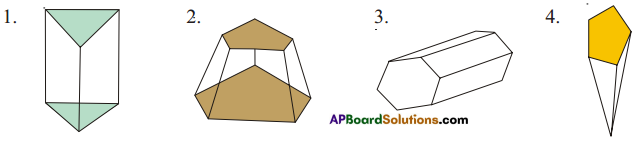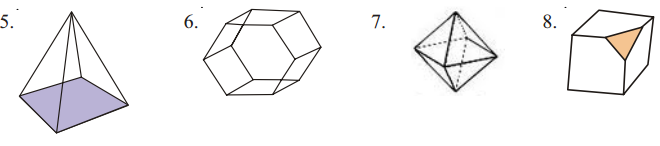Solution: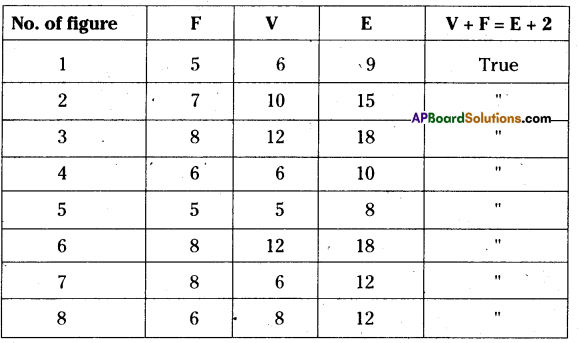Question 2.
Is a square prism and cube are same? explain.
Solution:
All cubes are square prisms, but converse is not true. (i.e.,) All square prisms are either cubes or, not.

Question 3.
Can a polyhedra have 3 triangular faces only? explain.
Solution:
Any polyhedra can’t have 3 triangular faces because the triangular pyramids are formed starts with 4 faces. So it does not exist.Question 4.
Can a polyhedra have 4 triangular faces only? explain.
Solution:
Yes, a triangular pyramid have 4 triangular faces.

Question 5.
Complete the table by using Euler’s formula.

 F 8 5 ? V 6 ? 12 E ? 9 30

Solution:

 F 8 5 20 V 6 6 12 E 12 9 30

i) E = V + F- 2 = 8 + 6- 2 = 12
ii) V = E + 2- F = 9 + 2- 5 = 6
iii) F = E + 2- V = 30 + 2-12 = 20Question 6.
Can a polyhedra have 10 faces ,20 edges and 15 vertices?
Solution:
No. of faces = 10
No. of edges = 20
No. of vertices = 15
According to Euler’s formula E = V + F – 2
⇒ 20 = 15 + 10 – 2
20 = 25 – 2
20 = 23 (False)
∴ A polyhedra doesn’t exist with 10 faces, 20 edges, 15 vertices.

Question 7.
Complete the following table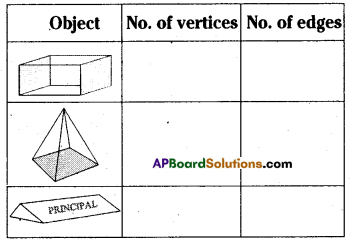Solution: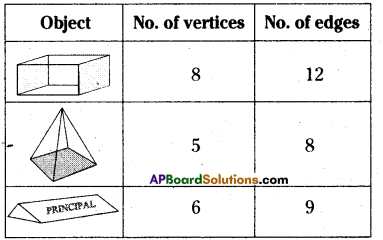Question 8.
Name the 3-D objects or shapes that can be formed from the following nets.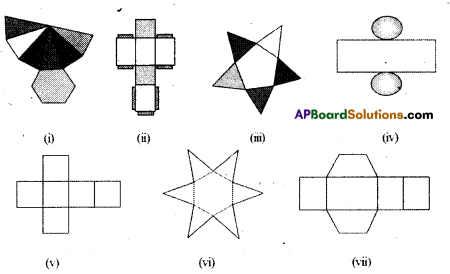(i) Hexagonal pyramid
(ii) Cuboid
(iii) Pentagonal pyramid
(iv) Cylinder
(v) Cube
(vi) Hexagonal pyramid
(vii) TrapezoidQuestion 9.
Draw the following diagram on the check ruled book and fmd out which of the following diagrams makes cube?
(i)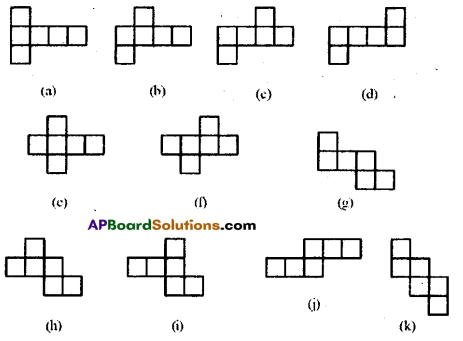Solution:
The diagrams which makes cubes are a, b, c, e.(a) Name the polyhedron which has four vertices, four faces’?
(b) Name the solid object which has no vertex?
(c) Name the polyhedron which has 12 edges’?
(d) Name the solid object which has one surface’?
(e) How a cube is different from cuboid?
(f) Which two shapes have same number of edges, vertices and faces?
(g) Name the polyhedron which has 5 vertices and 5 faces’?
Solution:
(a) Tetrahedron
(b) Sphere
(c) Cube/Cuboid
(d) Sphere
(e) Cube is a regular polyhedron where cuboid is not.
(f) Cube, Cuboid
(g) Square pyramid

(iii) Write the names of the objects given below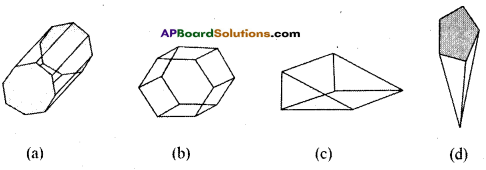Solution:
(a) Octagonal prism
(b) Hexagonal prism
(c) Triangular prism
(d) Pentagonal prism